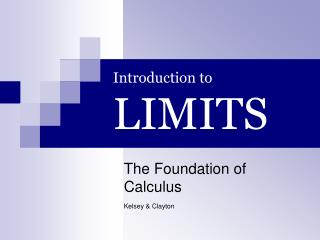Download PresentationIntroduction to LIMITS# Introduction to LIMITS - PowerPoint PPT Presentation

Download Presentation##### Introduction to LIMITS

Download Policy: Content on the Website is provided to you AS IS for your information and personal use and may not be sold / licensed / shared on other websites without getting consent from its author. While downloading, if for some reason you are not able to download a presentation, the publisher may have deleted the file from their server.

- - - - - - - - - - - - - - - - - - - - - - - - - - - E N D - - - - - - - - - - - - - - - - - - - - - - - - - - -
##### Presentation Transcript

1. Introduction toLIMITS The Foundation of Calculus Kelsey & Clayton

2. Limit: Basic Definition A Function’s Intended or Maximum Height Limits are the method of finding the maximum height, or y-value, a function can reach as x approaches a certain number. Limits calculate the y-value of a function that is usually unobtainable by using algebra. Because limits find the exact intended or maximum height of a function, they are useful in calculus and real-life situations where accuracy is critical.

3. Take a Look Here is the basic structure of a limit: The above limit is read as, “The limit of the function f (x) as x approaches c.” Basically, this means that as x approaches some constant, f (x) will eventually become something; f (x) will become its maximum or intended value.

4. Take a Closer Look Limit Anatomy 1. lim obviously stands for limit, indicating that the expression is, in fact, a limit. 2. f (x) is any valid function in calculus. For example, f (x) could be 2x + 2 3. x →c indicates the number x is approaching or is reaching its limit at. c can be any number. In this case c= 5, therefore x →5 (c can also be infinity and negative infinity: ∞&−∞)

5. A Family of Limits Limits come in all different shapes and sizes. Some functions have limits at all points, while others contain holes or asymptotes at different x-values. Some functions may not have a limit at all at a point because the function has more than one intended or maximum value. The previous problem was only a simple limit problem that could easily be solved by plugging in the c value for x in the equation. This method is an algebraic method. Other limit problems may require different methods to solve the limit such as graphing the limit or using numerical tables. Whatever the method required or whatever the type of problem, limits require a keen eye in order to determine how to evaluate a limit problem. Check out some of the sites at the end of this overview to practice your analyzing and problem-solving skills for limits.

6. The Foundation Limits are the fundamental building blocks of calculus. Without limits, neither derivatives nor integrals would exist. Derivative

7. Limits in Derivatives The above formula works the same way any other limit would work, except that the answer to the formula is used to find the slope at any point of the graph f (x). Using limits, you can find the exact maximum of f (x) when x approaches zero. This allows you to find the exact slope at a point when the change of x is at zero, something that would normally seem impossible with algebra.

8. Limits of Life "When will I ever use this in my life?" –Every Math Student's Favorite Question. One of the most useful applications of limits is in continuous compounding, a task too difficult for just algebra.  By using limits, you can easily create a formula that will continuously compound interest for a year.  Basically, the interest for a deposit would be compounded an infinite amount of times for an entire year.  Limits can easily create a formula that will automatically compound the interest an infinite amount of times over a span of one year. A = Pert

9. How do we obtain Pert? • Step 1: Pert is partially obtained when we take (1+(1/x))x as x approaches infinity. As we approach infinity, we obtain the number e which is 2.71828 approximately. • Step 2: We then use this when we take the limit of: P(1+(r/n))n as n approaches infinity. This lets us obtain Per which gives us the value after one year of continuous compounding. The formula Pert is used for when there are multiple years of compounding.

10. The Pebble “Snatch the pebble from my hand Grasshopper, and you may begin your true training.” –Some Zen Master Now that you’ve finished looking through this brief overview of limits, you may think you know all there is to know about limits. However, limits in calculus is a topic that can easily span ten chapters in a math book. Snatch the pebble from my hand and continue your limit training by visiting some of these links on limits. • http://www.karlscalculus.org/calc2.html • http://www.calculus-help.com/funstuff/tutorials/limits/limit01.html • http://mathforum.org/kb/search!execute.jspa?forumID=13&objID=f13&forceEmptySearch=true&q=limits • http://archives.math.utk.edu/visual.calculus/1/index.html

11. That’s All Folks!